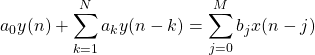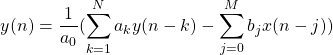# IIR Filter Implementation in F#

Source code for this implementation can be found here: https://github.com/BrothersRM/FsDsp

## Theory

In digital signal processing, one of the basic problems is the implementation of infinite impulse response filters. These are represented in the time domain by linear constant coefficient difference equations (LCCDE’s) of the following form:a and b are sets of coefficients by which current and previous values of the output and input signals, y(n) and x(n), are multiplied. By convention, a is 1. However, an a not equal to 1 applies a gain or attenuation to the overall result. Rearranging the above LCCDE a bit, we obtain the followingor, more directlyM and N are the number of b and a coefficients, respectively. b determines the zeroes of the resulting filter, while a determines the poles. Typically, M is less than N. When this is not the case, the filter is simply an IIR filter in series with some finite impulse response component. When N=1, the filter reduces entirely to a finite impulse response system, possibly with some gain or attenuation. This is also known as a zero-only filter. Likewise, setting M=1 creates a pole-only system.

Assuming N>M, N specifies the order of the filter. This determines how many previous values of x and y must be stored for computation. Consequently, this also determines how much memory our system requires. For embedded systems and FPGA implementations, this can cause problems. However, for the purposes of this example, memory consumption is not considered.

Put simply, every value of y(n) is determined by combining previous values of both the input and output with multiplicative constants.

The choice of values for a and b is critical, as it determines almost all of the behavior of the filter. However, it is beyond the scope of this post. Curious readers can consult Digital Signal Processing by Proakis and Manolakis for more information.

## Implementation

Digital filters are often thought of in terms of stages or pipelines. The output of one stage will be used as the input to the next. Naturally, this lends itself quite well to a functional style of programming. We might like to write code like the following, in pseudo F#

let output = input |> stage1.filter |> stage2.filter |> stage3.filter

This provides very high parity between the model of our filters and the usage in practice.

The implementation of fast filters demands some mutability in our choice of delay elements (IE, where we store previous values of x and y.

The delay elements exhibit behavior very similar to a queue. When we add a new element to the top, the oldest element is thrown out. A very straightforward implementation of this can be done with F#’s built-in linked-list

let newXState = f::xState.[0..M-1]

A simple benchmarking question to ask is “how quickly can we process one second’s worth of data”. For this implementation, I was interested in testing with audio data, which is commonly sampled at 44,100 Hz. To that end, I generated 44,100 float32 samples.

Benchmarking the above implementation yielded an average processing time of 10.032ms when using a fifth-order filter. While this is reasonable, there is a more efficient option: ring-buffers.

## Optimization

In order to optimize the performance of the filter, we can implement a simple ring-buffer data structure. This allows the data to be stored in-place, and allows for faster access of delay elements. This effect is particularly pronounced in higher-order filters.

My implementation of a ring buffer is specified below

let inline (%!) a b = (a % b + b) % b

type public CircularArray<'T> (a: 'T array ) =
let data = a
let mutable tracker = 0

data.[(tracker + index) %! a.Length]
member this.Write value =
if tracker = 0 then tracker <- a.Length - 1 else tracker <- tracker - 1
data.[tracker] <- value

member this.Item
with get(index) =
this.Read(index)

F#’s implementation of the modulo operator doesn’t do well with negative numbers, so I’ve used a modified version. This allows the -1st index of the array to wrap around to the end.

The tracker value is essentially a floating index, representing the head of the queue. When we write a new item to the queue, we simply advance the tracker, then write. Reading “starts” at the tracker, then uses modular arithmetic to determine the correct position within the array.

Benchmarking this implementation with the same data and setup yielded a processing time of 4.995ms, a speedup of slightly more than double. Full details of the benchmarking are listed below

In either case, the latency of each stage scales linearly with the order of the filter.

These tests were done using .NET 5.0. While they were performed in release-configuration, no additional optimization has been performed yet. With AOT compilation, processing times will likely be far faster.

The full implementation of the IIR class looks like this:

type public IIR (b: float32 array, a: float32 array, ?initialX: float32 array, ?initialY: float32 array) =
let N = Array.length a
let M = Array.length b

let _b = Array.indexed b |> Array.skip 1
let _a = Array.indexed a |> Array.skip 1

let xState = initialX
|> Option.fold (fun _ v -> v) (Array.zeroCreate M)
|> CircularArray
let yState = initialY
|> Option.fold (fun _ v -> v) (Array.zeroCreate N)
|> CircularArray

member public this.filter (data: float32 array): float32 array =
data |> Array.map (fun f ->
let sumB = _b
|> Array.fold (fun (sum: float32) (i: int, bi:float32) ->
(sum + bi * xState.[i - 1])) (f*b.)
let sumA = _a
|> Array.fold (fun (sum: float32) (i: int, ai:float32) ->
(sum + ai * yState.[i - 1])) (0.0f)
let yn = (sumB-sumA)
xState.Write(f)
yState.Write(yn)
yn
)


## Making it More Functional

This is a good implementation, but it isn’t particularly functional. To make it a bit more canonical, we can replace the implementation with closures, and modify the use of optional parameters.

let makeFilter (b: float32 array)(a: float32 array)(initX: float32 array)(initY: float32 array): (float32 array -> float32 array)=
let N = Array.length a
let M = Array.length b
let _b = Array.indexed b |> Array.skip 1
let _a = Array.indexed a |> Array.skip 1
let xState = initX |> CircularArray
let yState = initY |> CircularArray

let res (data: float32 array): float32 array =
data |> Array.map (fun xn ->
let sumB = _b |> Array.fold (fun (sum: float32) (i: int, bi:float32) -> (sum + bi * xState.[i - 1])) (xn*b.)
let sumA = _a |> Array.fold (fun (sum: float32) (i: int, ai:float32) -> (sum + ai * yState.[i - 1])) (0.0f)
let yn = (sumB-sumA)
xState.Write(xn)
yState.Write(yn)
yn
)
res

A second version of the function above, makeFilterZeroState, performs the same operation but doesn’t require the initial condition parameters. Instead, it initializes them to zero.

The performance of the above implementation is almost identical to that of the class-based version, but usage is slightly different. Instead of

let result = input |> stage1.filter |> stage2.filter |> stage3.filter

we have the comparatively simpler

let result = input |> stage1 |> stage2 |> stage3

This style also allows similar functions to be created somewhat more naturally, such as makeLowPass, makeChebyshev, makeButterworth, etc. Each filter requires and produces an array of 32-bit floating point numbers, and can thus be chained indefinitely, increasing only the latency of the system.

## Conclusions

The benefits of functional programming are usually not considered to be quantitative. Software engineers speak of maintainability, readability, resilience, elegance. They do not often speak of performance. To implement the real-time, high-performance functionality of a digital signal processing system requires certain sacrifices to be made, such as the use of a mutating ring buffer.

Compounding this, there is the inherently stateful nature of an IIR system; rapidly changing previous values are integral to the system itself, and each new value explicitly depends upon previous values of the same function. In other words, IIR systems are not referentially transparent unless previous values are passed in as a dependency, at which point they must also be computed and returned in addition to the output, rather than mutated in place. Without referential transparency, we do lose a key benefit of functional programming. The chained-dependency nature of IIR filters also prevents us from properly parallelizing many of internal operations of the filter.

However, the ease of composition and the use of higher order functions do provide a certain ergonomic benefit to the engineer. The code more naturally maps to the diagrams we draw and the models we create. Likewise, at the cost of performance, versions of these functions could easily be created that exhibit referential transparency. The goal of this experiment was to optimize performance while adhering as closely as possible to a functional paradigm, but a purely-functional approach may make an interesting project in the future.

One interesting problem to investigate will be the calculation of block-level initial condition results. As it stands, the filter cannot be truly parallelized at the block-level, as the final state of one block forms the initial conditions of the next block. A heuristic for calculating or guessing the N final results of one block would allow nearly-complete block-level parallelization, drastically speeding up the anticausal processing time. While not of great use in real-time applications, this would drastically speed up full-file processing. This will be investigated in a future project.

Tags: